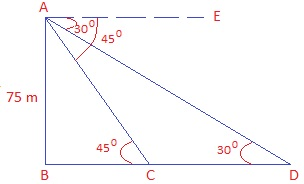Guru

# As observed from the top of a 75 m high lighthouse from the sea-level, the angles of depression of two ships are 30° and 45°. If one ship is exactly behind the other on the same side of the lighthouse, find the distance between the two ships. Q.13

• 0

What is the best solution for class 10th of ncert math of exercise 9.1 of question no.13 of Some applications of Trigonometry ncert, give me the best way to solve this problem in simple way. I think it is very important for class 10th board As observed from the top of a 75 m high lighthouse from the sea-level, the angles of depression of two ships are 30° and 45°. If one ship is exactly behind the other on the same side of the lighthouse, find the distance between the two ships.

Share

1. Let AB be the lighthouse of height 75 m. Let C and D be the positions of the ships.

30° and 45° are the angles of depression from the lighthouse.

Draw a figure based on given instructions:To Find: CD = distance between two ships

Step 1: From right triangle ABC,

tan 45° = AB/BC

1= 75/BC

BC = 75 m

Step 2: Form right triangle ABD,

tan 30° = AB/BD

1/√3 = 75/BD

BD = 75√3

Step 3: To find measure of CD, use results obtained in step 1 and step 2.

CD = BD – BC = (75√3 – 75) = 75(√3-1)

The distance between the two ships is 75(√3-1) m. Answer!

• 0